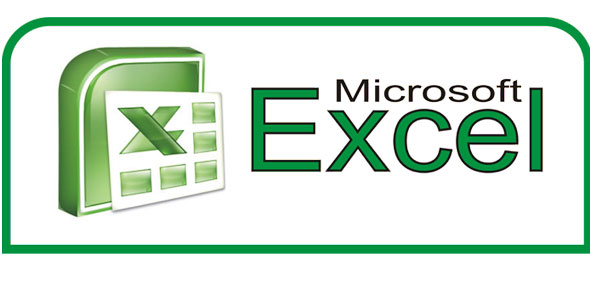# Excel - Pre Test

13 Questions | Attempts: 400
ShareSettings-

• 1.
Which view shows margins and the rulers?
• A.

Normal

• B.

Page Layout

• C.

Page Setup

• D.

Review

• 2.
Which function results can be displayed in AutoCalculate?
• A.

SUM and AVERAGE

• B.

MAX and AVERAGE

• C.

LABEL and AVERAGE

• D.

MIN and BLANK

• 3.
Which of these references is an example of a mixed reference?
• A.

B1

• B.

\$B\$1

• C.

\$B1

• D.

AB1

• 4.
How is a relative reference different from an absolute reference?
• A.

It includes column and row references.

• B.

It adjusts to its location on the worksheet.

• C.

It refers to an existing cell on the worksheet.

• D.

It resets to its original value.

• 5.
What category in the Format Cells dialog box is used to define a unique format for a date?
• A.

Custom

• B.

Date

• C.

Special

• D.

Text

• 6.
What statement describes this function: =SUMIF(D7:D14, ">1")?
• A.

It adds the values in cells D7:D14 if the value is greater than 1.

• B.

It adds all the negative values in cells D7:D14.

• C.

If the value in a cell is greater than 1, it is added to the other values in column D.

• D.

If the value in a cell is greater than 1, the range D7:D14 is placed on a summary sheet.

• 7.
Which of the following arguments in a financial function indicates the interest for a period?
• A.

FV

• B.

PV

• C.

Nper

• D.

Rate

• 8.
If a cell contains =ROUND(3.4567,2), what value would be used in a formula that refers to that cell?
• A.

3.45

• B.

3.46

• C.

3.457

• D.

3.4567

• 9.
Which of the following is the correct order for an A to Z sort?
• A.

1, 3, 5, 7

• B.

7, 5, 3, 1

• C.

• D.

May 1, 2007; April 1, 2007; March 1, 2007

• 10.
Which category of functions obtains data or displays information from the current or other workbooks?
• A.

Financial

• B.

Database

• C.

Lookup and Reference

• D.

Logical

• 11.
Which of the following statements about range names is false?
• A.

They are easier to remember than cell addresses.

• B.

They can be used for navigation.

• C.

They automatically substitute the relevant cell addresses.

• D.

They make formulas easier to understand.

• 12.
What is a macro?
• A.

Solver scenario

• B.

Visual Basic scenario

• C.

Visual Basic routine

• D.

Solver routine

• 13.
Why are PivotTables considered interactive?
• A.

They allow you to add dynamic data.

• B.

They allow you to rearrange row and column data.

• C.

They allow you to generate e-mail enabled reports.

• D.

They allow you to print the reports with various settings.

## Related TopicsBack to top
×

Wait!
Here's an interesting quiz for you.